# Team:Marburg/Model

Modeling

It is also a good rule not to put too much confidence in experimental results until they have been confirmed by Theory.
-- Sir Arthur Eddington

This wordplay by Sir Arthur Eddington highlights the importance of modeling in every discipline of science. Imagine bringing the predictability of physics, the exact measures of chemistry, and the rationality of maths into synthetic biology! The last decades have seen great strides towards this vision, but we still see potential for future improvement. We want to see this dream become a reality in the near future through modeling. Unlike the other sciences, biology has just begun realizing the vast potential of modeling.
With our model, we want to join a new generation of interdisciplinarity scientists working at, and exploring on the boundaries of what synthetic biology can deliver. With time nowadays being more precious than ever, we centred our project around saving it, streamlining processes and making these advances accessible to the research community.
We accelerated processes in many regards, but the most efficient way to save time is to know beforehand which experiments to conduct. The predictive powers needed for that can be harnessed by computer-based modeling. We harvested this prediction power with two independent modeling approaches, one predicting the metabolism of Vibrio Natriegens and the other designing an enzyme capable of a novel reaction, decarboxylating malate to 3-hydroxypropionic acid (3HPA).## Metabolic Model

In order to be able to fine-tune synthetic pathways and utilize the well-characterized parts of our cloning toolbox to its fullest potential, we need a model which gives useful predictions of metabolic fluxes. We decided to use a reaction kinetic based model and investigate the enzymes that we have the most control over – the ones that we introduce to the organism. With the help of our model we are able to obtain a detailed insight into how the concentration of Acc and Mcr change the product concentration. To improve the precision of our model, we also took the metabolic fluxes that the educt of our pathway is a part of into consideration. We applied this data to the Marburg Toolbox and calculated what the optimal promotors are for our metabolic engineering efforts. We could directly implement this data into our synthetic pathway to produce 3HPA.
Using the thereby consolidated theoretical knowledge we can complete the Design-Build-Test-Learn cycle for our metabolic engineering project and use it to iteratively improve our project. With the prediction power of our model, we not only save a lot of time in the lab but we manage to improve the efficiency and productivity, the skill ceiling of our pathway.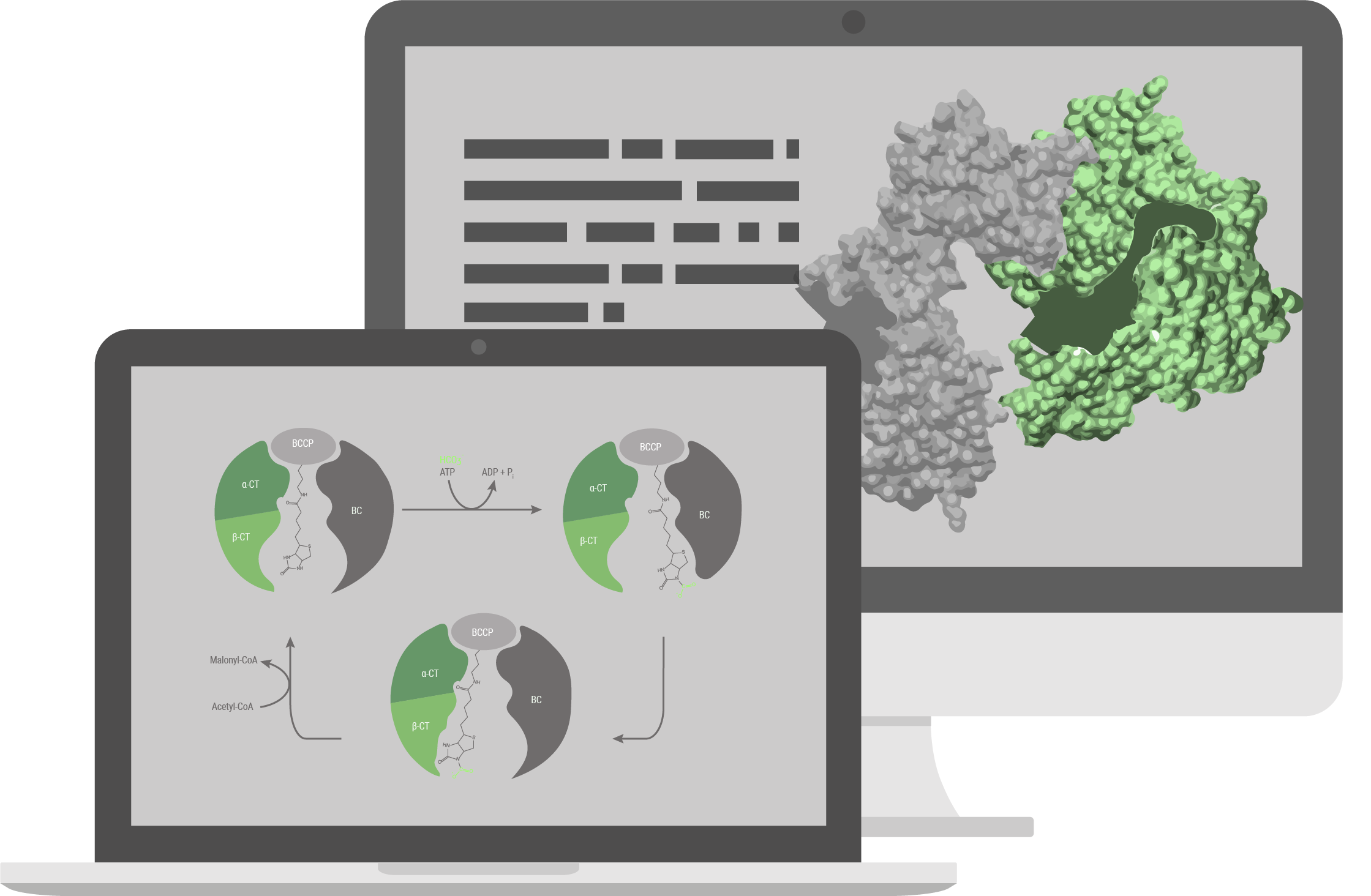## Structural model

The pathway we used for production of 3HPA (for details see the description part of the metabolic engineering subgroup) has been explored previously and is based on a combination of known reactions and known enzymes. Combination of different enzymes to make a synthetic pathway is a well-established method in the field of metabolic engineering, but limited to existing reactions and known enzymes. With our structural model, we tried to build a new energetically more favorable pathway which was previously impossible. To accomplish that, we needed to implement a reaction with no known enzyme to catalyse it, the decarboxylation reaction of malate to 3HPA. To build this pathway we decided to engineer an enzyme capable of catalysing this reaction. We investigated the enzyme family of Carboxy-lyases and developed an idea on how to build a binding pocket catalysing this reaction. To evaluate if our binding pocket works, we performed electronic structure calculations. With that, we were able to calculate the activation barrier of the reaction and therefore evaluate if it is possible for the reaction to take place. To advance from a binding pocket to a full enzyme we evaluated in silico mutated versions of acetolactate decarboxylase (ALD). For evaluating which mutants perform best we used MD simulations and checked how well the binding pocket assumed in the electronic structure calculations is represented in the simulations. With the help of these in silico approaches we chose a mutant and tested it in the wetlab.
Metabolic model

## Kinetics of the Enzyme Catalysed Reactions for our Pathway

### Motivation

For our pathway we need to introduce two new enzymes into V. natriegens.
In order to do this inteligently we must understand the mechanism which makes our pathway work - and for that we need to model its enzyme catalysed reactions and their response to variable changes.

Acetyl-CoA carboxylase (Acc) catalyses the irreversible carboxylation of acetyl-CoA to malonyl-CoA. Malonyl-CoA reductase (Mcr) catalyses two reactions, the reduction of malonyl-CoA to malonate semi-aldehyde and its reduction to 3-hydroxipropionic acid (3HPA). More about it can be learned here under metabolic engineering page.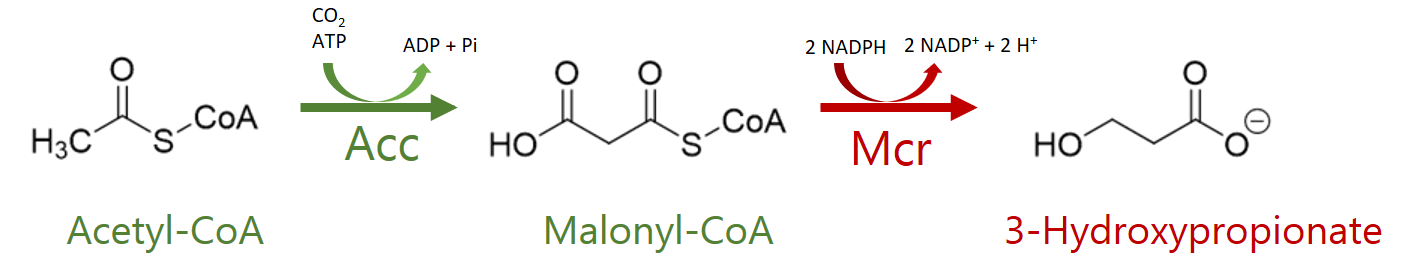Figure 1: The pathway we integrated into V. natriegens The red reaction is catalysed by Mcr - more about which can be learned here under Metabolic Engineering.
Foray into Reaction Kinetics

Reaction kinetics is the study of reaction rates of chemical processes. It investigates how different experimental conditions influence the speed of a reaction by transcribing that reaction's mechanism into a mathematical model.

There are many different experimental variables that have an impact on the reaction velocity as well as many possible mathematical representations of these impacts.
For example important for chemical reactions is the collision of the reactant molecules – which depends on their concentrations. To model this, one mathematically formulates the rate equations

in which the rate r of a reaction is given by the product of its substrate concentrations and a reaction specific factor k. There are different kinds of reactions which are denoted by the exponents x and y.
If a reaction mechanism is unknown one can experimentally determine reaction velocity and a rate constant for different substrate concentrations to acquire information about it.

Some reactions are however catalysed by enzymes not only dependant on the substrate concentration but also catalysed by enzymes.
One of the best-known models which describes the rate of such reactions is Michaelis-Menten kinetics.

To describe the rate of the enzymatic reactions Michaelis-Menten uses an equation relating the reaction velocity v to the concentration of the substrate as well as enzyme specific variables Km and kcat. Km is the substrate concentration at which the reaction rate is half of its possible maximum and has to be acquired experimentally – kcat is the catalytic rate – also acquired through experiment – that is the number of converted substrate molecules per enzyme molecule per second.

There are different possible mechanisms for enzyme kinetics.
Some incorporate reversible or irreversible inhibition - whereby the velocity of the Michaelis-Menten equations is moderated by the inhibitor concentration and an inhibition enzyme.
Other describe reactions with multiple substrates like the ternary-complex mechanism or the Ping-pong mechanism. In these the reaction rate is also dependant on the concentration of substrates and their reaction affinity with the enzyme. Multi-substrate reactions have additional Michaelis constants for the additional substrates.
If these constants are sufficiently small a multi substrate reaction can be approximated as a single substrate one.

We use Michaelis-Menten style reaction kinetics to describe the rate of the enzyme mediated reactions.
Most experimental enzyme measurements focus on getting the Michaelis constant Km. To use this we need the derivation of Michaelis-Menten kinetics which uses the quasi-steady-state approximation. This is the assumption that the concentration of the enzyme-substrate complex does not change whilst the reaction is going.

The equations of the reaction rates are Ordinary Differential Equations – these can be solved through integration to get the product concentration at all time points. For this we use Matlab – a numerical computing environment – which allows us to solve the ODE's and plot the solutions as well as write algorithms with which to analyse the model further.

### Methods

To start analysing our pathway we first need to know the Km and kcat values of the enzymes involved.

For Acc we encountered a lack of exact enzyme measurements within a sufficiently similar organism. However, we found ranges in which they could be expected. This would allow us to model the system and then put it through an error sensitivity analysis.

Table 1: Enzyme values used in the model
Enzyme Organism Km in μM kcat in s-1 Source
Acc E. coli 200 - 400 1 - 5 Fall et al. 1971
Mcr Chloroflexus aurantiacus 41.7 7.81 Rathnasingha et al. 2012
Rogers et al. 2016

For both Acc and Mcr we chose a single substrate reaction.

Purely reaction rate based Michaelis-Menten kinetics are derived using the law of mass action. This results for the single substrate mechanism four non-linear ODE's.

We decided to use the derivation through quasi-steady-state approximation. Most experimental measurements on reactions use the approach of measuring reaction velocity in dependence of the enzyme concentration. Hereby the Michaelis constant Km – with which the reaction velocity is half of the maximal velocity – as well as the catalytic constant kcat.

The initial and therefore maximal velocity of a reaction is a product of the initial enzyme concentration and the catalytic constant kcat which quantifies the number of catalysed product molecules by an enzyme molecule per time. This allows us to rewrite the equation.

So Km and kcat can then be used to make the velocity of a reaction only dependant on the enzyme and substrate concentrations. Here our resulting equations for both reactions:

Since we were not only interested in a qualitative but also a quantitative analysis, we needed to find realistic additional and secondary fluxes for the pathway we used for our metabolic engineering efforts in V. natriegens.
These fluxes into and from Acetyl-CoA were measured in V. natriegens by 13C labelled Metabolic Flux Analysis. (Long et al.2017)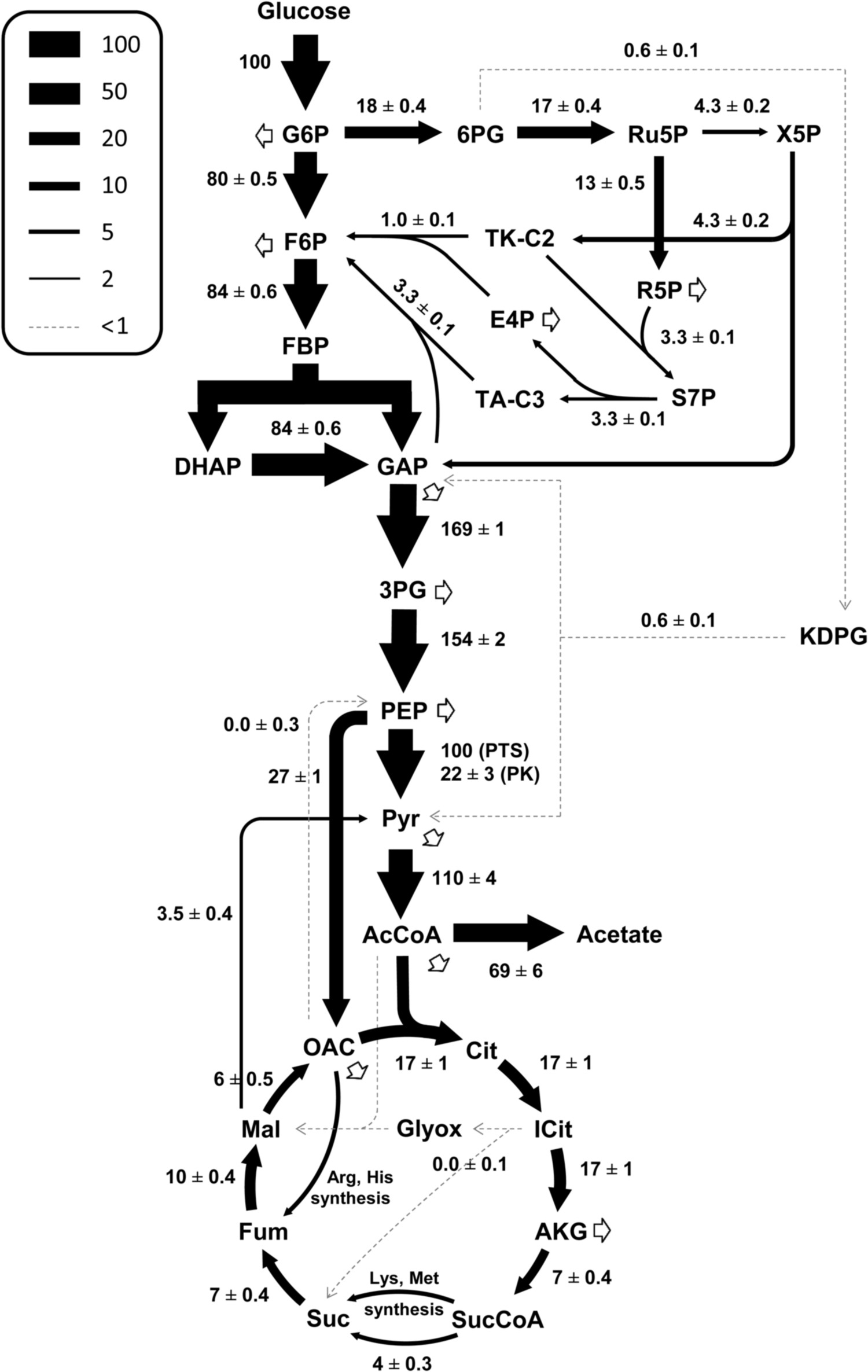Figure 2: 13C elucidated fluxes for the metabolism of V. natriegens (Long et al.2017)
13C elucidated flux data we use
Table 2:13C elucidated flux data important to us(Long et al.2017)
Reaction Normed to glucose uptake Flux in mmol/h/gDW
Glucose Uptake 100 21.4
Pyr -> AcCoA 110 23.5
AcCoA -> Acetate 69 14.8
AcCoA - > Cit cyclus 17 3.6

The exact Matlab scripts that we use can be found in dropdowns.

Basic Matlab Scripts
ODE's as a Matlab Function

function [dS] = Mechanism(~, S, E, K, k)

% Function containing reaction velocities
% meant to be integrated through ode15s or similar

S1 = S(1); S2 = S(2); S3 = S(3);
E1 = E(1); E2 = E(2);
K1 = K(1); K2 = K(2);
k1 = k(1); k2 = k(2);

v1 = (k1*E1*S1)/(K1+S1);
v2 = (k2*E2*S2)/(K2+S2);

% Secondary flows
F0 = 6.4;   %into Acetyl-CoA
F1 = 5;     %out of Maylonyl-CoA

dS1 = F0 -F1;
dS2 = +v1 -v2 -F2;
dS3 = +v2;

dS(1,:) = dS1;
dS(2,:) = dS2;
dS(3,:) = dS3;

end

Declaring Values

% Creates a structure array with the inputed values that is imported into
% other functions and scripts

S = [6.4 0 0]; % Substrate Conc.
E = [10 10]; % Enzyme Conc.
K = [400 41.7]; % Km
k = [5 7.8]; % kcat
tspan = [0 60];
options = odeset('NonNegative',1:3);

Values.title        = 'Values for KinMod SV';
Values.date         = datetime;
Values.S            = S;
Values.E            = E;
Values.K            = K;
Values.k            = k;
Values.tspan        = tspan;
Values.options      = options;
save('Values.mat','Values');

ODE solving and Production

% Calculates Production through time by solving Mechnism with ode15s

[t,y] = ode15s(@(t,y)Mechanism(t,y,Values.E,Values.K,Values.k), Values.tspan, Values.S, Values.options);

figure
plot(t, y);
xlabel('Time in s');
ylabel('Concentration in µM');
title('Simple Normed Version');
legend('AcetylCoA', 'MalonylCoA', '3HP');
set(legend,'Location','northwest');

Utility Functions
Variation of one variable

function [inputs, results] = VarOf1Var(Bounds,Scalation,Function,Values,Index)
%Variation of one variable in a ODE Function [Values manuel input still needed]
%{
Inputs different values for a variable into a ODE function and returns
a matrix of the results as well as imput scala
these can then be plotted against each other

Scalation = 0.1 means values looked at in 0.1 steps

Function gives ode solver as well as function it should solve
ODE function should be imputed with a "variable" in place of the variable to be
variied

Values struct file with other values the function uses, needs to be

Index is index of ODE return vector that needs to be looked at
this allows looking at different metabolites
%}

inputs = [Bounds(1):Scalation:Bounds(2)];
results = [];

for i = inputs
Y = ODE(i);
%results(1,i) = Y;
results = [results Y];
end

function [Y] = ODE(i)
variable = i;
[tout,yout] = eval(Function);

N = [tout,yout];
Y = N(end,Index);
end

end

Variation of two variables

function [inputs1, inputs2, results] = VarOf2Var(Bounds1,Bounds2,Scalation1,Scalation2,Function,Values,Index)
%Variation of one variable in a ODE Function [Values manuel input still needed]
%{
Inputs different values for a variable into a ODE function and returns
a matrix of the results as well as imput scala
these can then be plotted against each other

Scalation = 0.1 means values looked at in 0.1 steps

Function gives ode solver as well as function it should solve
ODE function should be imputed with a "var1" in place of the first
variable to be variied
var1 is row index var2 is column inedx

Values struct file with other values the function uses, needs to be

Index is index of ODE return vector that needs to be looked at
this allows looking at different metabolites
%}

inputs1 = [Bounds1(1):Scalation1:Bounds1(2)];
inputs2 = [Bounds2(1):Scalation2:Bounds2(2)];

%{

Could add following to chose between bounds and single values
needs finishing

if length(Bounds1) == 2
inputs1 = [Bounds1(1):Scalation1:Bounds1(2)];
else
continue

if length(Bounds2) == 2
inputs2 = [Bounds2(1):Scalation2:Bounds2(2)];
else
continue

%}

results = [];

for i = inputs1
Yrow = [];
for j = inputs2
Y = ODE(i,j);
Yrow = [Yrow, Y];  %Yrow is row vector of column variable outputs
end
results = [results; Yrow];
end

function [Y] = ODE(i,j)
var1 = i;
var2 = j;
[tout,yout] = eval(Function);

N = [tout,yout];
Y = N(end,Index);
end

end

Getting 95% of leveling value

function [x] = FindXforLevel95(Y,s)
%   Takes Vektor Y and gives index x for which value is 95% of last value
%   in vector
%   also takes index scalation s to give back real value and not index for
%   when index isn't real value

level = Y(end);
level95 = level/100*95;
l = length(Y);

for i = 1:1:l
if Y(i) >= level95
x = i./s;
break
else
continue
end
end
end

We used matlabs intern stiff differential equation solver ode15s to integrate the ODE's over a chosen period of time.
This mechanism we implemented into Matlab functions and scripts and its solution builds the basis of all further analysis.

With this we are able to elucidate the kinetics of our pathway while being able to vary things like enzyme concentration or the Km and kcatvalues of the enzymes. The production of 3HPA was calculated for a time period of 60 seconds for normalisation purposes and to limit the computational demand.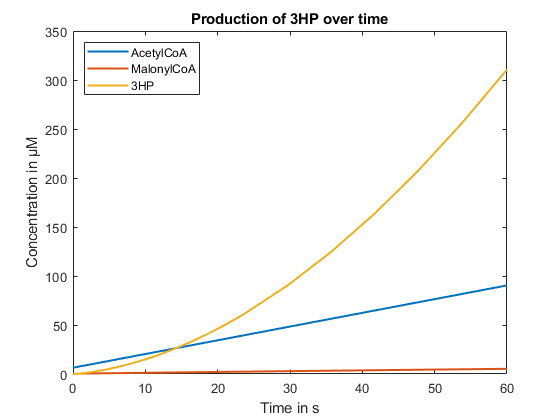Figure 3: In the above figure we calculated the production of 3HPA over a period of 60 seconds. The Acc and Mcr were both at 10μM Shown are also the concentrations over time of Acetyl-CoA and Malonyl-CoA.

### Enzyme Concentration and Optimal Enzyme Ratio

The first thing that we investigated to optimize our pathway is the dependence of the production of 3HPA to the expression levels of the enzymes.
To investigate the effects resulting from the changes in Acc and Mcr concentrations we calculated the velocity of each reaction for various concentration values.

Matlab scripts for the variation of the enzyme expression
Variation of both enzyme concentrations

%{
Value for 3HPA is taken 60 sec after Reaction begins
Other variables are taken from Values.mat by loading it and then using the
structure array called Values and giving it on to VarOf2Var
%}

%%  Getting Matrix
Bounds1 = [0 100]; %acc in µM
Bounds2 = [0 50]; %mcr in µM
Scalation1 = 1;
Scalation2 = 0.1;
Function = 'ode15s(@(t,y)Mechanism(t,y,[var1 var2],Values.K,Values.k), Values.tspan, Values.S, Values.options)';
Index = 4; %3HPA %Index of resulting vector, can be used to "sample" different substances from it

[acc_scala, mcr_scala, results] = VarOf2Var(Bounds1,Bounds2,Scalation1,Scalation2,Function,Values,Index);

%%  Getting 95%Level Points

%{
results consists of (i,j) where i is acc and j is mcr -> results(acc,mcr)
surf() uses [y, x] = size(Z) - so you need results.' (.' is transpose)

95% points of mcr in loop over acc row vectors from results(acc,mcr)
%}

mcr_points = [];
index_mcr_points = [];
index_acc = 1:1:length(acc_scala);
for i = index_acc
index_mcr = 1:1:length(mcr_scala);

index_mcr_point = FindXforLevel95(results(i,index_mcr),1);
%index_point corresponds to index after which mcr is > 95% of level
index_mcr_points =  [index_mcr_points index_mcr_point];

mcr_point = mcr_scala(index_mcr_point);
mcr_points = [mcr_points mcr_point];

end

points = nan(length(index_acc),length(index_mcr));
for i = index_acc
point = results(i,index_mcr_points(i));
points(i,index_mcr_points(i)) = point;
end

%%  Plotting Results

figure('Name','EE variation')
hold on % Allows for plot3 to be in same figure
s = surf(acc_scala,mcr_scala,results.'); % Plots a surface
title('3HPA by enzyme expression variation');
view(-45,30); % Sets point of view
colorbar; % Adds a colorbar beside the figure
s.EdgeColor = 'none'; % Removes net from the plot surface
xlabel('acc in µM');
ylabel('mcr in µM');
zlabel('3HPA in µM')

%Plot Points
plot3(acc_scala,mcr_points,points,'o','Color','k');

%%  Linear fiting of Points and resulting formula and plot of it

P = polyfit(acc_scala,mcr_points,1);
yfit = P(1)*acc_scala + P(2); % Gets Slope and y-Intercept from polyfit
figure('Name','Linear fit')
plot(acc_scala, yfit,'r-.')
title('Linear fiting of saturation points');
str = {'Slope = '+string(P(1)), 'y-Intercept = '+string(P(2))};
ylim = get(gca,'ylim');
xlim = get(gca,'xlim');
text(0.25,0.75,str,'Units','normalized') % Positions text
xlabel('acc in µM');
ylabel('Saturation point of mcr in µM');

Variation of one enzyme concentration whilst other is held

% Single Enzyme Expression

%3HPA Value taken 60 sec after Reaction begin
%The other enzyme expression is held as given in Values struct

%% acc with held mcr

Bounds = [0 1000];
Scalation = 1;
Index = 4;
E_held = [10 25 50 75 100 150 200];

figure('Name','varied acc concentration with held mcr concentration')
for i_E_held = E_held
hold on
Function = 'ode15s(@(t,y)Mechanism(t,y,[variable '+string(i_E_held)+'],Values.K,Values.k), Values.tspan, Values.S, Values.options);';
[inputs, results] = VarOf1Var(Bounds,Scalation,Function,Values,Index);
plot(inputs, results);
end
title('3HPA by acc concentration with mcr concentration held (legend)');
xlabel('acc in µM')
ylabel('3HPA in µM')
legend(string(E_held));

%% mcr with held acc
Bounds = [0 50];
Scalation = 0.1;
Index = 4;
E_held = [10 25 50 75 100 150 200];

figure('Name','varied mcr concentration with held acc concentration')
for i_E_held = E_held
hold on
Function = 'ode15s(@(t,y)Mechanism(t,y,['+string(i_E_held)+' variable],Values.K,Values.k), Values.tspan, Values.S, Values.options);';
[inputs, results] = VarOf1Var(Bounds,Scalation,Function,Values,Index);
plot(inputs, results);
end
title('3HPA by mcr concentration with acc concentration held (legend)');
xlabel('mcr in µM')
ylabel('3HPA in µM')
legend('10', '25', '50', '75', '100', '150', '200');

In Figure 4 we can observe the production of 3HPA for different Acc concentrations with a fixed mcr concentrations. We can see that for every Mcr concentration there exists a point after which more Acc doesn't produce more 3HPA. At this saturation point Mcr becomes a rate limiting step.

In Figure 5 we can observe the production of 3HPA for different mcr concentrations with a fixed acc concentration. In this figure we also notice even more clearly that there exists a saturation point after which more Mcr doesn't increase the 3HPA production and Acc becomes the rate limiting step.
The saturation point is reached much earlier than before, indicating that we need more acc expression than mcr.

As can be observed in Figure 4 and 5 more Acc than Mcr is needed. This means that Acc is the prime rate limiting step of 3HPA production.

Furthermore we looked at the points of saturation of Mcr after which a stronger expression of it would be negligible for better production. For this we utilize the points at which Mcr reaches 95% of the value that it levels at. We then plotted these saturation points as can bee seen in Figure 6. We performed a linear regression on these data points to determine their relation.

The slope of this linear regression corresponds to the Mcr concentration divided by the Accconcentration. We concluded that the ratio of enzyme expression of optimal efficiency is situated along this line and is 0.10416. We can then use this in the next step.

### Comparison of Relative Promoter Strengths

Our Part Collection team has developed the Marburg toolbox featuring many different promoters.
This well characterized toolbox made it possible for the metabolic engineering team to fine tune the enzyme expression ratios with the help of different promotor combinations.
For this we needed to investigate which promoter combinations would lead to the best 3HPA production.

We needed to look at the optimal ratio (without looking solely for best prodcution) of the promoter pairs – so as not to burden the cell metabolism unnecessarily through the expression of uneccesary enzymes – as well as get the promoter combination with the highest possible production. To find a good balance these then needed to be combined to lessen the metabolic burden whilst preferably still keeping a high production.

To find the optimal ratio of promoter usage the point of saturation for Mcr after which its increase doesn't lead to a noticeable increase in 3HPA production was used. It was assumed that the ratio of the expression of Acc and Mcr at this point is optimal.

The promoters are ordered from the strongest, J23100, whose relative strength has been normed as 1, to the weakest J23103. A representation of every relative strength can be found here.

Figure 8 shows all possible ratios relative similarity to the optimal. It has been normed to between 0 and 1. The values on the z-axis have been plotted on a logarithmic scale with exponent 100 so as to get a better sense of the scale broadness as well as to clearly recognise the best combination.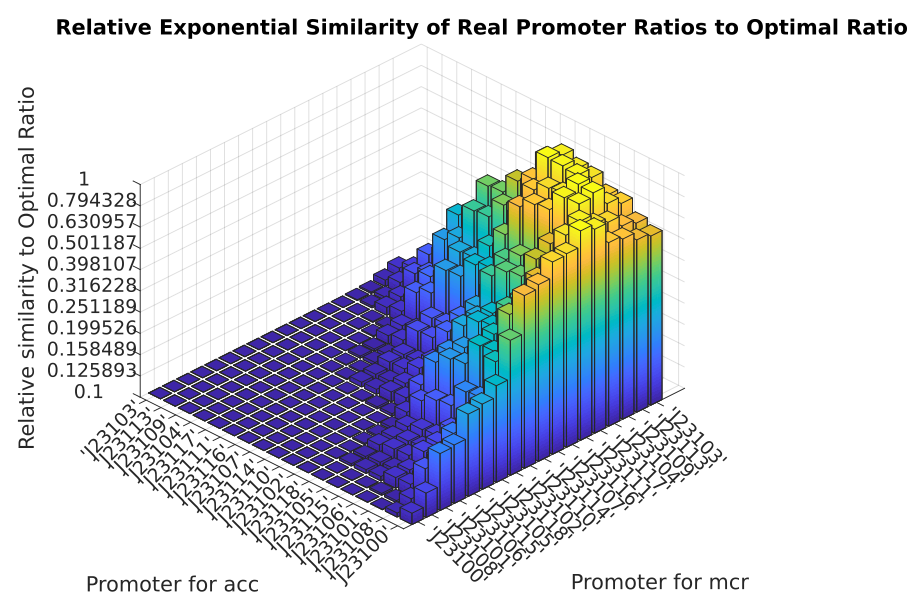Figure 8: Combinations of the relative promoter strengths have been compared to the optimal ratio

For a theoretical optimal ratio of 0.10416 the best promoters are J23106 for Acc and J23109 for Mcr with 97.981% similarity to the optimal ratio.

### Relative Promoter Production

Since we only knew the relative strengths of promotors and no absolute values we do not know at which concentration the enzymes would be expressed. The outcome of the model is changing with the order of magnitude at which the enzymes are expressed. To investigate this we attributed different real enzyme concentrations to the normal promoter strengths. Hereby we chose to associate the strongest promotor with different decimal magnitudes of enzyme epression and therefore concentration. It has been assumed that the same promoter would have the same expression for Acc and Mcr. And thus no combinations of different orders of magnitude have been shown.

As can be observed in the Graphs for 100nM as well as 100μM – for very small and very big concentrations – there occur boundary effects.
These are caused by the fluxes to and from Acetyl-CoA as well as the Km and kcat values of both enzymes. Due to the similarity in the Figure for 1μM and Figure 10μM we concluded that all concentrations between the ones investigated are not influenced by boundary conditions.
This range without effects from boundary conditions is not exhaustive and due to limited time we did not investigate it fully.

Since physiological enzyme concentrations also lie within the lower μM concentrations we strongly believe that our model is a reasonable approximation.

### Impact of Acc variables

Since we couldn't find trustworthy Km and kcat values for Acc – only a range within which the real ones probably are – it was important to look at the impact of the resulting error on the model.

For this reason we first looked at the impact of the simultaneous variation of Acc values for Km and kcat on the 3HPA production. Every 3HPA value was taken at 60 seconds from reaction begin for the solutions of ODE's containing the corresponding values on the axes.

For the scripts we used to determine these changes click below:

Matlab scripts for the variation of the Km and kcat values for Acc
Variation of both values

% Single Enzyme Expression

%3HPA Value taken 60 sec after Reaction begin
%The other enzyme expression is held as given in Values struct
%EE held for both at 10 µM

%% acc with mcr values held

%%{

Bounds1 = [200 400]; %Km in µM
Bounds2 = [0 5]; %kcat in s^-1
Scalation1 = 10;
Scalation2 = 0.1;
Function = 'ode15s(@(t,y)Mechanism(t,y,Values.E,[var1 Values.K(2)],[var2 Values.k(2)]), Values.tspan, Values.S, Values.options)';
Index = 4; %3HPA %Index of resulting vector, can be used to "sample" different substances from it

[acc_scala, mcr_scala, results] = VarOf2Var(Bounds1,Bounds2,Scalation1,Scalation2,Function,Values,Index);

figure('Name','acc Km and kcat variation for held enzyme expression')
s = surf(acc_scala,mcr_scala,results.'); % Plots a surface
title('3HPA by acc Km and kcat variation for held enzyme expression');
view(-45,30); % Sets point of view
colorbar; % Adds a colorbar beside the figure
s.EdgeColor = 'none'; % Removes net from the plot surface
xlabel('Km in µM');
ylabel('kcat in s^{-1}');
zlabel('3HPA in µM')

%}

Variation of one value whilst other is held

%%  acc with variation in only one

%%{

%   Km
Bounds = [200 400];
Scalation = 10;
Index = 4;
kcat_held = [1 2 3 5];

figure('Name','acc Km variation for different kcat')
for i_kcat_held = kcat_held
hold on
Function = 'ode15s(@(t,y)Mechanism(t,y,Values.E,[variable Values.K(2)],['+string(i_kcat_held)+' Values.k(2)]), Values.tspan, Values.S, Values.options);';
[inputs, results] = VarOf1Var(Bounds,Scalation,Function,Values,Index);
plot(inputs, results);
end
title('3HPA by acc Km variation for different kcat (legend)');
xlabel('acc Km in µM')
ylabel('3HPA in µM')
legend(string(kcat_held));

%   kcat
Bounds = [0 5];
Scalation = 0.1;
Index = 4;
Km_held = [200 250 300 400];

figure('Name','acc kcat variation for different Km')
for i_Km_held = Km_held
hold on
Function = 'ode15s(@(t,y)Mechanism(t,y,Values.E,['+string(i_Km_held)+' Values.K(2)],[variable Values.k(2)]), Values.tspan, Values.S, Values.options);';
[inputs, results] = VarOf1Var(Bounds,Scalation,Function,Values,Index);
plot(inputs, results);
end
title('3HPA by acc kcat variation for different Km (legend)');
xlabel('acc kcat in s^{-1}')
ylabel('3HPA in µM')
legend(string(Km_held));

%}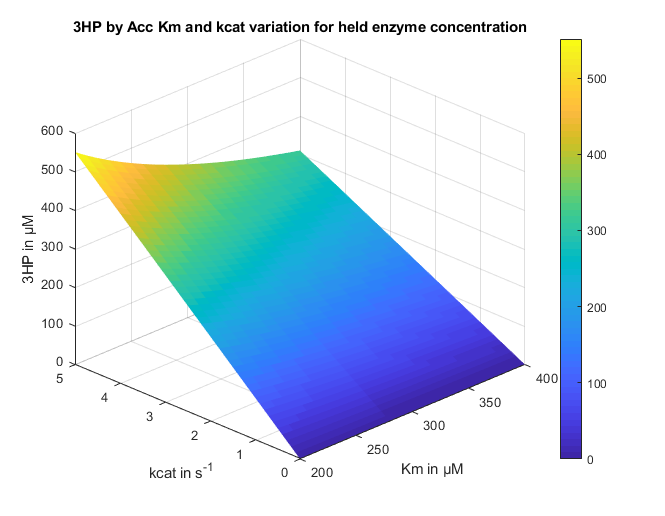Figure 10: 3HPA production for the simultaneous variation of Km and kcat values of Acc

The bounds for Km and kcat which were plotted have been chosen as the likely ranges for the values as mentioned above.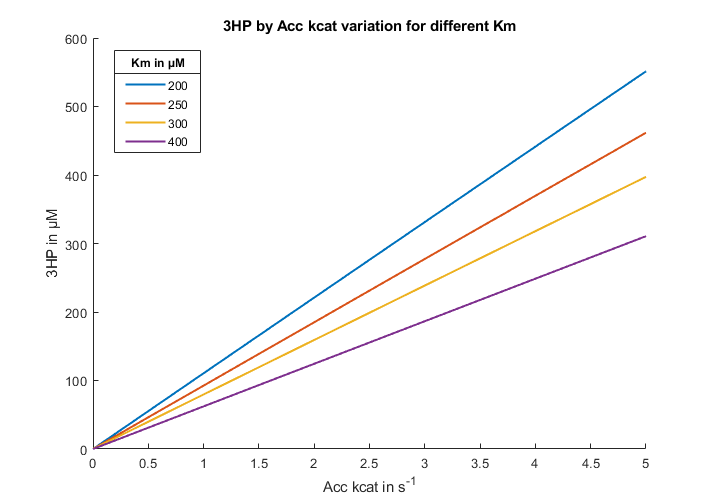Figure 11: Lienar 3HPA production dependancy on the kcat value of Acc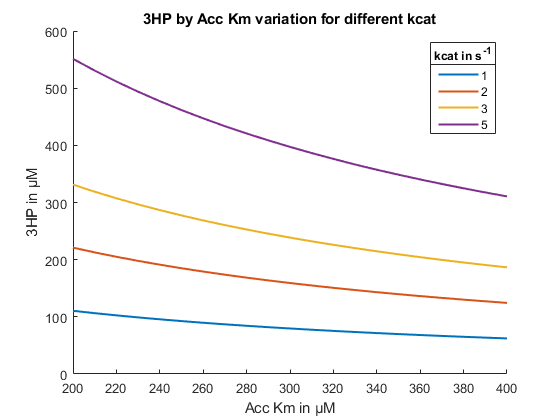Figure 12: Logarithmic decrease of 3HPA production dependant on the Km value of Acc

In figure 11 we are only changing the value of kcat to exactly how the model responds. Hereby we can observe linear increase in total 3HPA production.

In figure 12 we have looked at the variation of Km whilst kcat is constant. The change of the Km value causes a logarithmic decrease of total 3HPA production. Furthermore this decrease is greater with increasing kcat. This exacerbates the sensitivity of the model which is caused by kcat alone. The model is thus much more sensitive towards a change in kcat as opposed to a change in Km. Which means that it is more important for us to have a good approximation of Acc kcat values.

### Summary

With our metabolic model we investigated the enzyme kinetics of the pathway that we introduced into V. natriegens. Hereby we took into account all pathways that lead to and from Acetyl-CoA, the starting point of our pathway.

We examined the effects of enzyme concentration on the pathway and discerned that Acc is the prime rate limiting step and that there exists a linear relationship between Acc and the Mcr saturation points.

With this, we were able compare the relative promoter strengths characterised in our Marburg Toolbox to find the best promoter combination.
Additionally we looked at results of possible real enzyme concentrations for the relative promoter strengths.
Hereby we ascertained that promoters J23106 for Acc and J23109 for Mcr had the best ratio of enzyme expression.

Furthermore we investigated the effects of uncertainty in the values of Acc and found that kcat has the highest impact on changes in the 3HPA production.

This is where our project showed its full potential because we were able to use the well-characterized Marburg Toolbox and calculate which promotor combination yields the most expression of 3HPA.

### Outlook

To be able to increase the accuracy of our model, we need to improve the accuracy of the enzyme specific constants that we feed it with.
For this we need to calibrate the model with real measured concentrations.

To do this we implemented a biosensor with which to measure the production of 3HPA as well as Malonyl-CoA. More about it can be learned here under Metabolic Engineering.

The bio sensor data can be used to measure production across time and this data can be used to first see which values for Acc are most compatible and then also be used to maybe look for better fitting mechanisms for the enzyme kinetics.

Going a step further we planed on adding a Metabolic Flux Analysis using a novel method which combines Flux Balance Analysis and 13C labelled Metabolic Flux Analysis.
With this method, we would not only be able to observe how well our pathway works, but also how our pathway influences the metabolism of the whole cell. This would allow us to make statements with high accuracy not only how to make the best possible pathway to produce 3HPA, but also how to do this in a way that is feasible for the organism.

Structural model

## Teach the rabbit to quack Expanding the scope of metabolic engineering through novel enzyme engineering

### Infrastructure

We want to thank Prof. Kolb and Prof. Klebe for kindly providing us with the necessary infrastructure and software access to perform our calculations. We also want to thank the Marc-2 and the HRZ for the computational resources. For our QM calculations, we used the program GAUSSIAN09 and Chemcraft to set up our systems. For a foray to how these kinds of calculations work, please click below.

Foray to QM Calculations

### Foray to QM Calculations

QM Calculations are the pinnacle of precision compared to all other methods used for calculating molecular systems, but this precision comes with very high computational costs. All QM calculation try to solve the Schroedinger Equation :

They do that by calculating the Hamiltonian of the system. The Hamiltonian is an operator that if applied to the wavefunction of a system results in the same wavefunction times its energy. QM calculations are characterized by two important things: the functional and the basis set used. The functional is characterized by the way the Hamiltonian is calculated. For all calculations that we do, we also need a starting point to describe orbitals or the density function (we will go into more detail to this later) and the basis set describes the number and type of the orbital functions that we use to calculate the system.

### Born Oppenheimer Approximation

A necessary assumption to use QM methods is the Born Oppenheimer Approximation. This is the approximation that due to the vast difference in speed between the electrons and the nucleus of the atoms the movement of both can be investigated apart from each other. For QM Methods this means that we only look at the movement of electrons and neglect the very slow movements of the nuclei when solving the Schroedinger Equation.

### Functionals

There are two fundamentally different types of functionals, wave function based and density function based methods. We only used density function based methods, to be precise B3LYP. Density Function Theory (DFT) is centered not on the wavefunction but on the square of the wave function, i.e. the electron density. It is based on the theorem of Hohenberg and Kohn and modern DFT is also based on the Kohn Sham approach. The electron density is a measurable quantity that is dependent on the cartesian coordinates of the electrons. In the first days of DFT (when invented by physicists) mostly the Linear Density Approach (LDA) was used and this approach works fine for metallic solids, in which the electrons are evenly distributed. However, this is a poor approximation when we want to model molecular systems. As a further Development of this approximation, Gradient corrected methods (GGA) have been invented. These methods consider the electron density not to be uniform.
There are multiple terms in the Hamiltonian that have to be calculated. The most troublesome one is the so-called "Exchange-Correlation" term. There is a class of DFT functionals, the so-called Hybrid Functionals, that use a combination of exchange-correlation terms of different methods. The method we used, B3LYP, uses a combination of LDA, GGA, and Hartree Fock (A wavefunction based method) exchange-correlation.

### Basis Sets

Now that we know which method we use we can take a look at the second important characteristic of our QM calculations, the basis set. All methods described previously, even if based on DFT, use Orbitals in their calculations. By combining a certain number of orbitals the molecular orbitals of the system have to be expressed. This starting number of orbitals is called the basis set. Obviously, the more orbitals we have the more accurate the calculations become, but they also get more demanding. As a small basis set we used the 6-31g* variant and as a bigger basis set, we used cc-pVDZ. In a perfect scenario, the use of a bigger basis set should only improve the accuracy of the result, but it is possible that using a different basis set yields completely different results.

### Summary

• Based on Quantum Mechanics
• All electrons investigated
• Bond cleavage and formation can be calculated
• Slow Calculations and limited to small Systems
To perform MD Simulations we used the program package AMBER16. For a foray how MD Simulations work, please click below.
Foray to MD Simulations

### Foray to Molecular Mechanics

Molecular Mechanics (MM ) uses the laws of classical mechanics to model molecular systems. It can be used to calculate anything ranging between small molecules to big proteins. Each atom is simulated as one single particle, with a radius and charge. Bonded interactions are treated by the famous Hooke's law (i.e. they are springs). This approximation allows us to simulate very big systems, but it is very important to asses which properties we can simulate correctly and therefore investigate. Because classical Molecular Dynamics (MD) Simulations are based on MM , we cannot simulate bond breaking or bond formation which also means that protonation states cannot change during simulation.

### Force Fields

To calculate inter- and intramolecular interactions, so-called force fields are used. With these FF all of these interactions are reduced to single additive terms. It consists of terms for bond stretching, angle bending, torsions, Lennard-Jones potential (for Van-der-Waals interactions) and electrostatic potential. It is necessary to parametrize these terms very carefully and this is done by using experimental data or QM calculations. There are many FF available and we used a force field from the AMBER family called ff14SB (Maier et al.2015).
The complete potential function is given in the following equation:

### Kinetic Energy and Time Evolution of a molecular system

If we would just apply all of the physical principles and assumptions mentioned previously, we would minimize the system to the closest potential energy minimum. For the system to overcome potential energy barriers given by the FF, we have to introduce kinetic energy. The kinetic energy of all particles in the system is dependent on their temperature and given by a Maxwell-Boltzmann velocity distribution function.

This distribution function contains the kinetic energy of a particle, which is expressed as its momentum.

The total energy of the system is given as the sum of the potential energy (given by the force field) and the kinetic energy. Each particle in every MD timestep that we investigate has, therefore, three positional coordinates, three momentum coordinates, and a corresponding total energy.

To now introduce time evolution in the system we use classical mechanics and newtons laws of motion in combination with the kinetic energies introduced previously.

To further reduce the computational cost a so-called leapfrog algorithm is used. As mentioned previously, every particle in the system is described using three positional coordinates, three momentum coordinates, and its corresponding energy. With the leapfrog algorithm for each step of the MD we only calculate either the positinal coordinates or the momentum coordinates and "leap over" the other.

### Periodic Box

MD Simulations are performed within a confined volume, called a unit cell. This unit cell contains all particles that we simulate (i.e. protein, water, ligand). This introduces the problem of boundary effects because atoms and molecules close to these boundaries of the unit cell have fewer interaction partners than those in the middle of it. To avoid boundary effects at the edges of the unit cell we repeat the unit cell periodically. Thus, the shape of the unit cell has to allow such that a regular space filling lattice of unit cells can be arranged. In our study, we used a truncated octahedron.

### Summary

• Based on Classical Physics
• No Electrons investigated
• No Bond cleavage or formation
• Fast Calculations and big Systems

### Motivation

We went to great lengths to develop a workflow for metabolic engineering that utilizes the power of directed evolution and is worthy of synthetic biology in the not so early 21st century. With the very recent Nobel Prize in chemistry towards directed evolution we think we have struck a nerve, and with Vibrio Natriegens this process can be streamlined even further. Traditional Chemistry with Synthesis as its supreme discipline and its implications in the industry is one of the driving forces behind the modern wealth and is being improved on a daily basis. Synthetic Biology and in particular metabolic engineering as a chemical producing science can use that well-established knowledge and expand on it. Due to the completely different approach to synthesizing chemicals, we can mend the problems that classical organic chemistry is facing (e.g. natural products with many stereocenters, necessary purification of products after most reaction steps) and create compounds previously not synthesizable (e.g. Artemisinic acid) or ones that were too costly. Using metabolic engineering also has the advantage to produce chemicals out of renewable resources while many chemicals right now are synthesized starting with fossil oil. To further improve this opportunity to make more of the chemical space synthesizable in a cheap, easy and renewable manner we need to go beyond "mix and match" pathways and explore novel enzymatic reactions. According to (Erb et al.2017) metabolic engineering has been categorized in 5 different levels, depending on the methods employed. These levels and the corresponding metabolic space is displayed in Figure 1. We tried to expand on what we already did in our metabolic engineering project and tap the huge advantages using novel pathways offer. We tried to enable a novel pathway by engineering an enzyme to catalyse a new reaction which corresponds to the 4th level of metabolic engineering.Figure 1: Different levels of metabolic engineering and their respective biochemical space.(Erb et al2017)

### Design of the binding pocket

The pathway we chose for our metabolic engineering efforts was - in our opinion - the best pathway that has been explored previously. But there are much more possible theoretical (Valdehuesa et al,2013) pathways with remarkable properties that have not been explored yet. The reason is most of the times that it involves one or more reactions with no known enzyme to catalyse them. One theoretical pathway is from a free energy standpoint much more favorable, but there is one step without a known enzyme to catalyse it. We performed an intense literature research and decided to build an enzyme capable of decarboxylating malate to 3HPA. Even with Vibrio natriegens if we would engineer an enzyme to catalyse the wanted reaction using random mutagenesis it would take ages if it succeeds at all. That is why we decided to use a combination of in silico as well as wet lab methods to boost our chances of succeeding.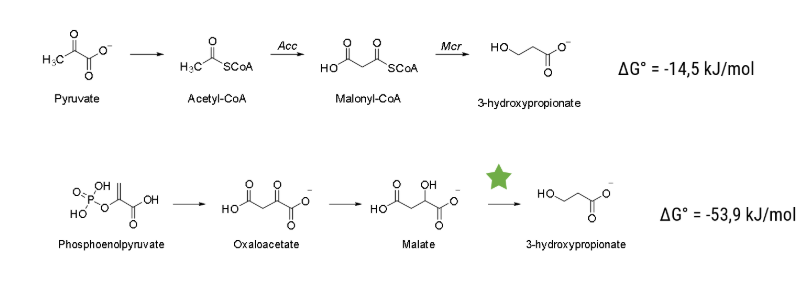Figure 2: Comparison between established and theoretical pathway using free energy. Reaction with no enzyme to catalyse marked with a green star.

The pathway that we plan to enable is displayed in Figure 2. The step that has to be catalysed is the decarboxylation reaction of malate to our final product, 3HPA. As a starting point, we investigated the enzyme family of Carboxy-lyases (EC Number 4.1.1). We - in accordance with literature - were not able to find an enzyme that can catalyse this reaction. However, there were some that we thought could help us to develop a binding pocket capable of decarboxylating malate.
One of those was acetolactate decarboxylase (ALD 4.1.1.5). The important difference from its natural substrate to malate being that there is a carboxy group in β position (see Figure 4). In this enzyme with the help of this carboxy group a double bond is formed to an intermediate product (see Figure 4) that we cannot form with malate as substrate. But the zinc cation that is used as a cofactor should be able to bind to malate in a similar way as it does with the natural substrate. This could be a promising starting point because we would be able to sustain a specific conformation of the substrate and alter the electronic structure of the substrate at the same time. The complex of malate inside the ALD binding pocket is shown in Figure 3. The zinc cofactor is bound by three histidines and the zinc binds to the malate (or the substrate analog in the real crystal structure) with three interactions. There is another important residue close to this complex, Arg145. After the double bond and with it the intermediate product is formed, this residue is protonating the intermediate to form the product.Figure 3: Complex of Malate with the Zn Cofactor inside the ALD binding pocket [PDB 4BT3]. .

Another enzyme with an enzyme mechanism that might help us to find a way to catalyse this reaction is Orotidine 5'-phosphate decarboxylase (ODCase 4.1.1.23). The reaction ODCase catalyses compared to the reaction of ALD and the one we want to catalyse is shown in Figure 4.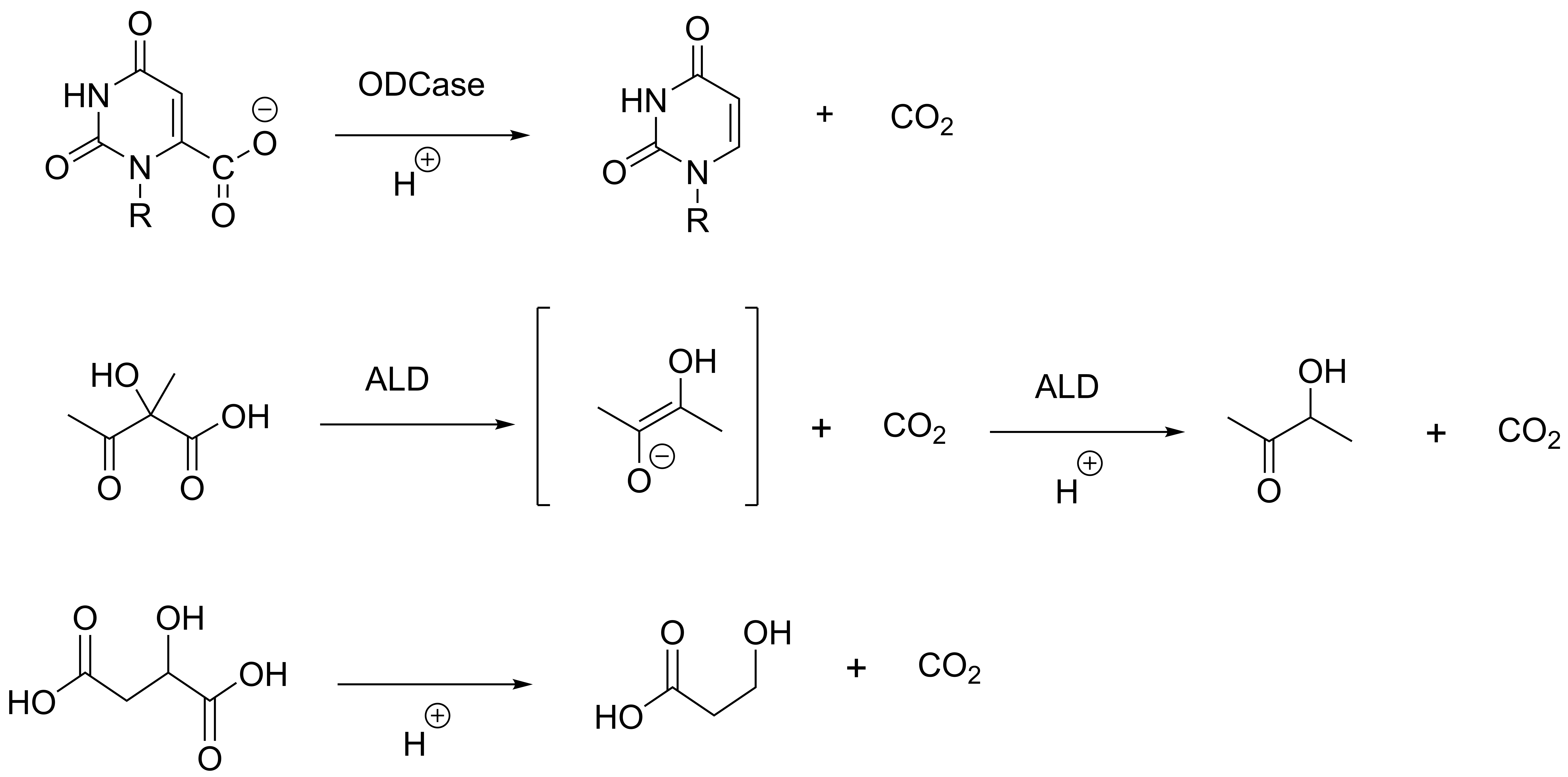Figure 4: Comparison between the reaction catalysed by ODCase , ALD and the reaction that we want to catalyse. .
In one study conducted by (Courtney et al.2007) using QM/MM MD simulations, the decarboxylation mechanism of ODCase has been investigated. They have proposed a direct decarboxylation mechanism and calculated a free energy activation barrier that is in good agreement with experimentally obtained values. The reaction is split into two steps, first the decarboxylation and a simultaneous salt bridge between a lysine and the resulting carbanion. After this, the lysine protonates the carbanion to yield the final product. The most important difference between the substrate of ODCase and malate is, that the carbanion stabilized (Figure 4) in the former one whilst there is nearly no stabilization in the latter one. The idea of our enzyme design is that we use the direct decarboxylation of ODCase and stabilize the carbanion with the cofactor of ALD. With this plan, we need to engineer the pocket in a way that a lysine side chain can get to the C2-carbon of malate (see Figure 5 for numbering) that shall be protonated.Figure 5: Carbon chain numbering of malate (left). Highlighted bonds around which the dihedral angle 1 (red) and dihedral angle 2 (green) are centered (right). Even if we can place a lysine near to the substrate, the reaction is not automatically working since the transition state energy is probably too high. We need to calculate the transition state energy of our engineered systems and compare it to the literature to estimate the feasibility of our reaction. If the reaction is not feasible with a single lysine in the binding pocket, we need to alter the binding pocket to lower the transition state energy. After that, we need to mutate an enzyme to resemble the binding pocket.

As a starting point, we looked at all single point mutations to lysine that can be done where the protonated nitrogen of lysine has the possibility to get as close to the malate since this is required for reprotonation. All Mutations investigated are displayed in Table 1. In the natural binding pocket of ALD there is an arginine residue that is used for reprotonation of the natural substrate. Because of the possibility that this disturbs the lysine we mutated it in each binding pocket where it is not already mutated to a glycine.

Table 1: All point mutations to ALD (PDB 4BT3) that have been investigated. The aminoacids are displayed as single character with the residue number in between. All mutations besides R145K also include R145G.
L34K G57K T58K L62K
E65K G64K R145K V147K

### in silico enzyme design

We developed a double modeling approach to investigate the whole system. First, we investigated the reaction using quantum mechanical calculations (QM) and model the best possible system. Then we control how well this system is retained if we make certain mutations in the ALD with the help of molecular dynamic (MD ) Simulations.

### Quantum mechanics calculations

First, we modeled the reaction that we plan to catalyse using QM level calculations. We used density functional theory based method b3-lyp with different basis sets. With these calculations, we tried to get a further insight into the reaction coordinate and all corresponding energies, most importantly the activation energy of the reaction. The activation energy can be calculated as the difference between the energy of the starting compounds and the transition state energy. The activation energy is crucial for the activity of the final enzyme and high activation energies correspond to low or no activity of the final enzyme. According to (Courtney et al.2007) the reaction is split into two steps. First, the decarboxylation happens and a salt bridge is formed between the positively charged lysine and negatively charged carbanion. In a second step one of the protons of the lysine is protonating the negatively charged carbanion and with that the reaction is complete. The first step of the reaction is the rate-limiting step of the reaction (Courtney et al.2007) and because of this, we decided to focus on it.

#### Building of binding pockets

As a starting point we used the ALD crystal structure of (Marlow et al.2013) (PDB CODE 4BT3). From that, we extracted the position of the three zinc coordinating histidines, the zinc cation, and the substrate and changed the substrate analog ((2R,3R)-2,3-Dihydroxy-2-methylbutanoic acid) to malate. We then built multiple different binding pockets that are displayed in Table .

Table 2: The differently setup systems with the changes made and names given.
Type of Binding Pocket Abbreviation used in Graphs Residues/Molecules involved
Binding Pocket without Cofactor Complex Lysine, Substrate
Binding Pocket Nor_1 Three Histidines, Zinc cation, Lysine, Glutamate, Substrate
Binding Pocket pre-optimized Nor_2 Three Histidines, Zinc cation, Lysine, Glutamate, Substrate
Minimal Binding Pocket Min Three Histidines, Zinc cation, Lysine, Substrate
Binding Pocket with two lysine residues 2lys Three Histidines, Zinc cation, Lysine, Lysine, Substrate

Because we want to resemble the binding pocket to the best of our knowledge we made a system in which we kept Glu253 and added a lysine close to the C2-carbon that it should protonate. Later we used a pre-optimized structure of previous calculations and used this pre-optimized structure to build a minimal binding pocket where we removed Glu. We also made one system with two lysine residues. We will go into detail on why and how we designed this system when we explain the hypothesis. As a start point, we also used a structure of malate and lysine, referred to as complex in Table 2. The final systems are displayed in Figure 6.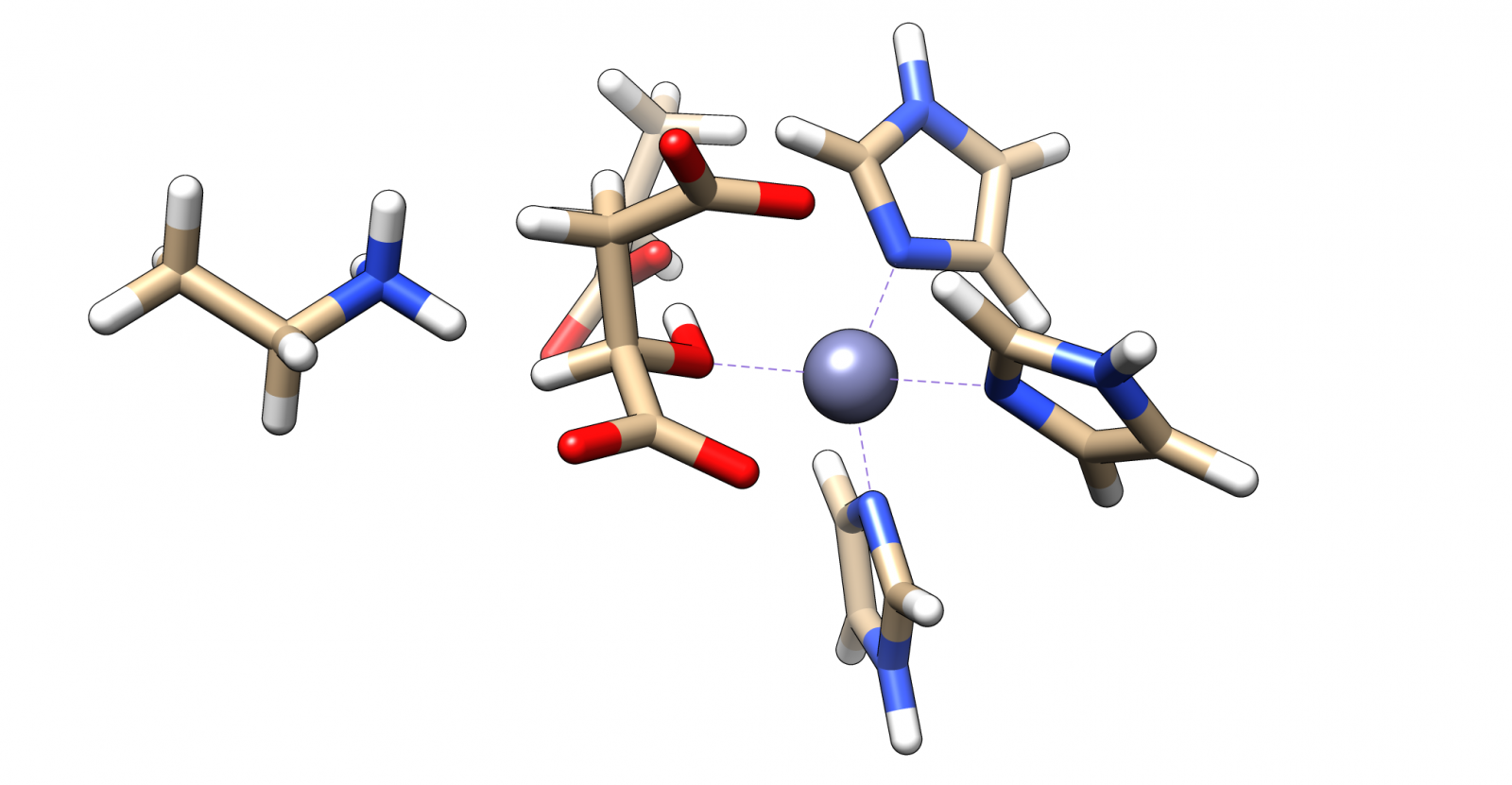Figure 6 : Displayed of the different binding pockets. a) pre converged full binding pocket b) post converged full binding pocket c) minimal binding pocket d) binding pocket with two lysine residues To evaluate the transition state energy and the overall energy profile of the reaction an investigation of the reaction path is necessary. Since we investigated a decarboxylation reaction, which is a c-c bond break, we chose to set up multiple calculations at different c-c bond lengths to evaluate the energy necessary to split that bond. This way we sample the reaction coordinate of the systems. We screened the bond length in 0.1 Angstroem steps and added between 0 and 2 Angstroem to the starting c-c distance, this results in 20 calculations per screen. With this method, we performed calculations for the starting system (no added c-c distance), the transition state (varying c-c distances depending on the system) and the final product (high c-c distances).

#### Results of QM Calculations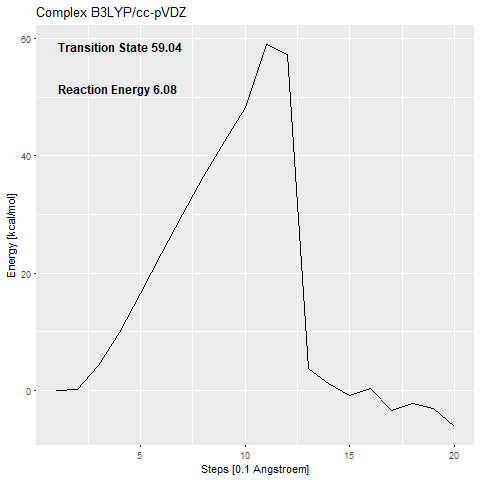Figure 7: Energy Profiles of C-C bond lengthening The results of the calculations can be seen in Figure 7.
The calculated activation barrier for the complex (cc-pVDZ basis set) is 59.04 kcal/mol [Figure 7a]. This is far over the free energy barrier of ODCase in the literature (15.54 kcal/mol (Courtney et al.2007)). The addition of the zinc complex that shall stabilize the carbanion (53.48 kcal/mol) [Figure 7c] only benefits the reaction with around 6 kcal/mol. The transition states that we calculate are confirmed using frequency calculations. If there is just one negative frequency calculated for an optimized structure, this means that it is a transition state. The frequency can then be animated, to show the movement that is enforced by it. An animation of the negative frequency of the minimal binding pocket transition state can be seen in Figure 8.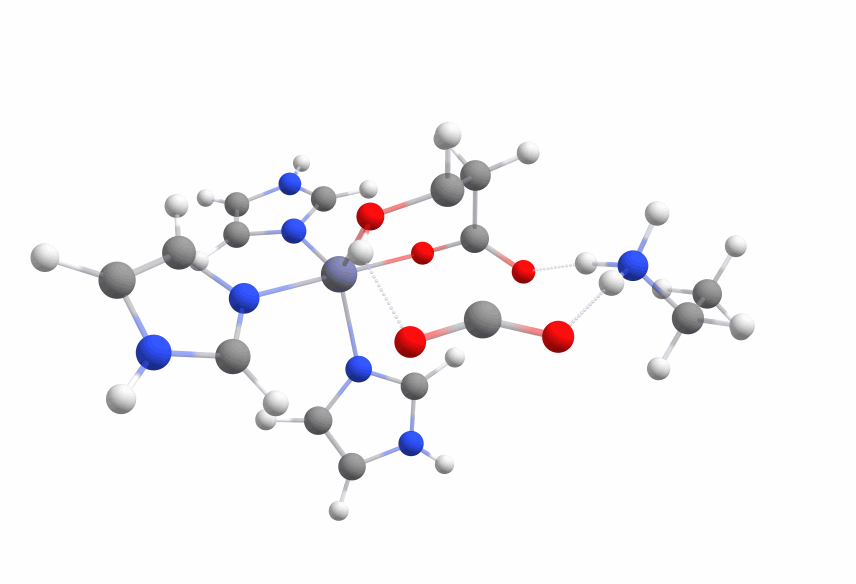Figure 8: Animation of negative frequency of the transition state of the nor_1 binding pocket. Since all electronic structure calculations conducted in this study are computationally quite demanding, starting structures were pregenerated by structure optimizations on a low level of theory. The optimized structures are then used to build the starting structures for high-level calculations on the normal binding pocket and the minimal binding pocket. As geometric optimizations are heavily dependent on the starting structure, we got different results with these structures than we got previously. The Nor_2 (cc-pVDZ basis set) [Figure 7b] and the min (6-31g* basis set) [Figure 7d] electronic structure calculations were set up using a pre converged structure. For these two calculations, the activation barrier was approximately 7 kcal/mol less than in the previous calculations. This was due to the fact that the lysine was not in the intended place at the end of the minimization. Rather than building the salt bridge with the carbanion, it was interacting with both carboxy moieties, which was lowering the activation energy.

This was boosting our hope that if we introduce the correct changes to the binding pocket, we could change the electronic structure of malate in a way to sufficiently reduce the activation barrier. To use this knowledge we set up another system with two lysine residues, one interacting with both carboxy moieties (lys1) and one that shall protonate the C2-carbon (lys2). The activation barrier (2lys 631g*) was slightly lower than before (~ 43 kcal/mol), but lys2 was not reprotonating the carbanion but interacting with the hydroxy moiety. Even more interesting was the fact that the carbanion formed a covalent bond with the zinc cation with ca. 10 kcal/mol less energy than the transition state. The optimized structure with the covalent bond between the zinc cation and the malate is displayed in Figure 9.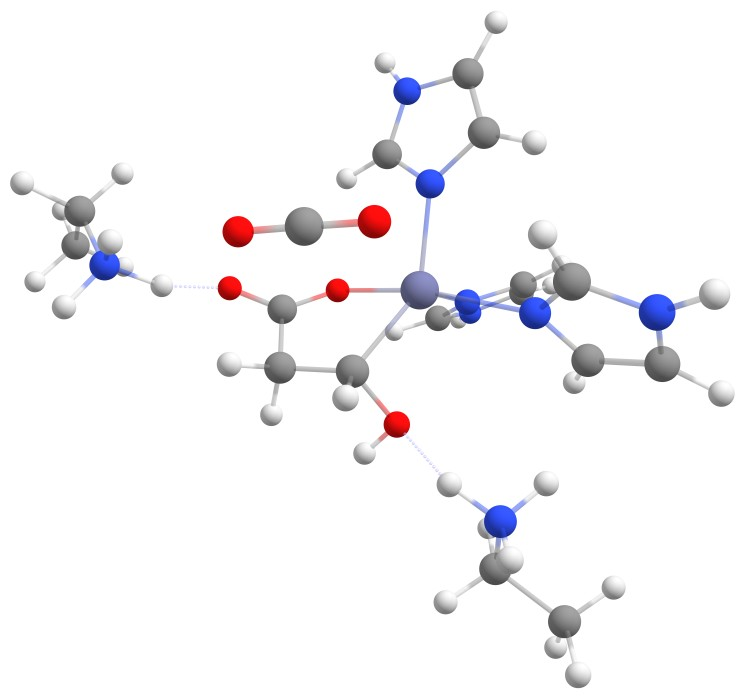Figure 9: Intermediate state of the reaction with covalent bond between zinc cation and malate. This adds a new way at which the reaction can take place that can be used as an alternative approach without the need of direct reprotonation.

### Molecular Dynamic (MD ) Simulations

To evaluate how well the system that we tried to engineer in the QM calculations is represented in the complete enzyme systems we performed MD simulations with in silico mutated enzyme versions. We performed 200 ns MD Simulations of all previously mentioned mutated systems as well as the wild-type enzyme with 3 replicas each. With the help of these MD simulations, we are able to evaluate how well the different mutations resemble the system that we designed for the QM calculations.

#### Setup of MD Simulations

For the MD simulations, we used the ALD crystal structure of [Reference] (PDB CODE 4BT3). We changed the substrate to malate and made the corresponding mutations for each system. Then we capped the termini of the enzyme, checked for missing residues and protonated all residues according to pH 7.0. We visually inspected the residues close to the binding pocket to make sure that all protonation states are correctly assigned. For a more detailed description of our preparation protocol please click below.

MD preparation protocol
Crystal structures of ALD were prepared using MOE. Ligand partial charges are derived using AM1-bcc calculations. The programs antechamber and parmchk2 are used for assignment of gaff atom types and missing force field parameters. Then tLEaP is uesed to assign ff14SB (Maier et al.2015) force field parameters to the protein and counterions (Na+, Cl-) are added to ensure charge neutrality. After building the truncated octahedron TIP3P-Ew water box, parameter and starting structure files are saved to disk.
In the following, all minimization steps are carried out using pmemd and all MD runs are carried out using pmemd.cuda from the AMBER 16 package. Before the MD run is started, we first heat and equilibrate the system. For that, we minimize the system first with fixed solute heavy atoms and afterwards with constrained solute heavy atoms. The system is annealed to 300K within 25 ps. After that, the density is equilibrated to approximately 1g/ml within 25 ps while lowering the constraints on the solute heavy atoms. A final 5 ns equilibration with fixed volume and temperature is carried out before starting the productive MD.

#### Results of MD Simulations

We previously established the mutations we introduced in silico (Table 1) to ALD. For evaluating how well the mutants resemble the binding pocket developed with the help of the QM we developed a mechanical descriptor. The distance between the binding pockets lysine side chain and the malate is used. We measured the distance between the lysine sidechains nitrogen atom and the C2-carbon that should be protonated of the malate for every frame of every MD Simulation. This way we can evaluate if the lysine is close enough to the C2-Carbon for reprotonation as well as interaction with the carboxy moieties. An animation of one MD Simulation (L34K Replica 1) with the distance highlighted is shown in Figure 10.

To display this data we chose to use normalized histograms. This way we can display the variation of this one variable. Normalization means that we do not display the number of observations on the y-axis, but rather the density of observations in each bin. The total area of the histogram is normalized to one. This helps to compare different histograms. We also colored the three replicas that we simulated for each mutated enzyme differently, so that we can compare the different replicas. The data is displayed in Figure .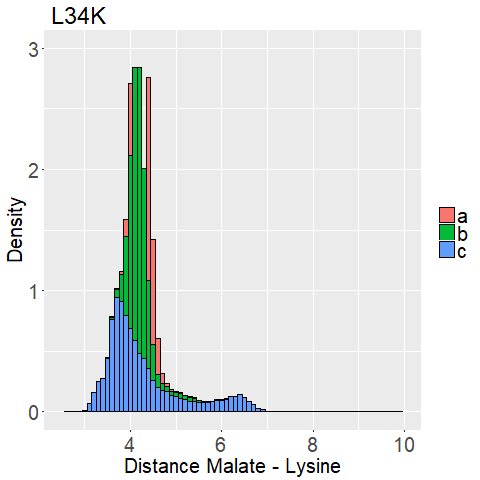Figure 11: Histograms of all simulated trajectories. Histograms colored differently for each replica a) L34K enzyme mutant b) R145K enzyme mutant c) V147K enzyme mutant d) L62K enzyme mutant e T58K enzyme mutant f) E65K enzyme mutant The first thing that stood out to us is that all histograms show mostly low distance ( < 6 Angstroem) and most replicas (besides E65K_c [Figure 11e] and R145K_b [Figure 11c]) are in good agreement to each other. Apart from R145K and V147K all variants show distances under 4 Angstroem. Especially L34K, T58K, and E65K have stable and low distances.
This is very promising because it means that there is not only one potential position to introduce point mutations, which opens up further possibilities for enzyme design. To further distinguish between the different variations we decided to look at two dihedral angles of the lysine sidechain to evaluate its flexibility. To display this we used two-dimensional histograms. The 2d histograms we developed contain all the information the 1d histograms had. However, they do not "just" count how many instances of an investigation we have in a bin, but rather how many combinations of investigations we have in a small two-dimensional box. This way we can not only investigate which distances each mutated enzyme has, but also pair this together with the corresponding dihedral angle(s) [Figure 5]. We also use normalized 2d-histograms, which means that the total area of the 2d-histogram is normalized to one. What we are looking for is a mutated enzyme which consistently shows low lysine-malate distances while simultaneously showing some flexibility in the dihedral angles. If the side chain of lysine shows too much flexibility (i.e. Figure 13d), the sidechains stability at the correct position is unfavorable. A ligand that shows too low flexibility (i.e. Figure 12b) the position is entropically unbeneficial. The results are displayed in Figure 12 and 13.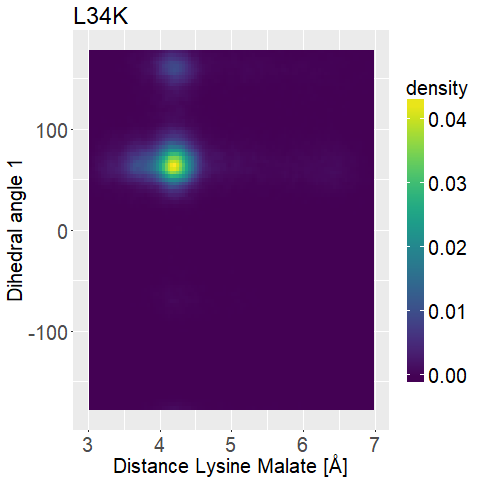Figure 12:2D Histogram Lysine-Malate Distance vs Dihedral angle 1. a) L34K enzyme mutant b) R145K enzyme mutant c) V147K enzyme mutant d) L62K enzyme mutant e T58K enzyme mutant f) E65K enzyme mutant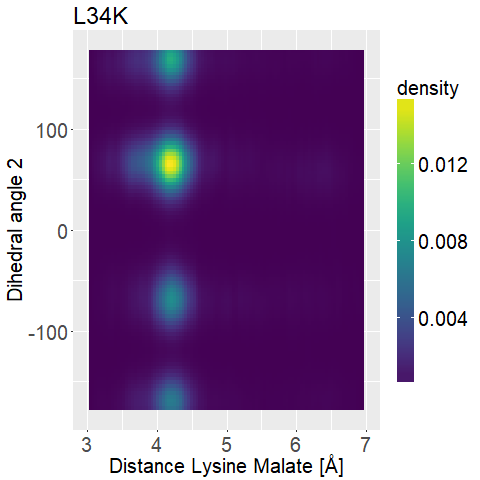Figure 13: 2D Histogram Lysine-Malate Distance vs Dihedral angle 2. a) L34K enzyme mutant b) R145K enzyme mutant c) V147K enzyme mutant d) L62K enzyme mutant e T58K enzyme mutant f) E65K enzyme mutant

We can observe high densities for R145K (Figure 12b), which means that the single state it is in is also very stable. The very stable dihedral angles indicate an entropically unbeneficial conformation of the side chain. For T58K we observe a stable conformation at a low distance, but also that it is stable at a broad range of distances. L34K has multiple stable angles for dihedral angle 2, whilst having only one for dihedral angle 1 and a small and low distance range. If we take the 1d as well as the 2d histograms into account, L34K seems to be the most promising of all of the different mutated enzymes.

### Wetlab

Even though our QM calculations did predict that the mutated enzyme won’t show activity, we decided to test this hypothesis in the lab.
For validation we planned to express it in V. natriegens. ALD is an enzyme involved in mixed acid fermentation, a metabolism which is widespread in the domain of bacteria. To lower the risk of implementing an enzyme which isn’t functional in our strain, we decided to use the ald gene of an organism closely related to V. natriegens. We decided for ald from Enterobacter cloacae ATCC 29006, because it is a model organism for studying mixed acid fermentation, is also a γ-proteobacterium and has biosafety level 1. We codon-optimized the gene sequence of the L34K ALD mutant for V. natriegens and ordered it.
Our plan was to clone it into an expression vector and transform it into our chassis. By using the 3HPA biosensor (See our metabolic engineering biosensor part for more details) we then can visually see if 3HPA is produced.
To quantify the amount of produced 3HPA we planned to perform plate reader measurements detecting fluorescence of GFP/RFP or luminescence, triggered by the lux operon, depending on which reporter is used. To further analyze enzyme activity, we planned to perform a coupled enzyme assay, where we overexpress the protein and break the cells to get cell extract.
As described in the section about product screening (See our metabolic engineering product screening part for more details) cell extract can be used in a photometric assay together with an NAD(P)H-dependent reaction to quantify substrate conversion. In this case we decided to use propionyl-CoA synthase (PCS) from Chloroflexus aurantiacus as indicator enzyme. PCS catalyzes the NADPH-dependent conversion of 3HPA to propionyl-CoA. The reaction mix consists of ATP, MgCl2, CoA, NADPH and cell extract of ALD L34K producing cells.
To start the reaction, we add malate and by determining the slope we then can calculate the specific activity. Figure 14 illustrates the route, to measure NADPH consumption by ALD and Pcs. To confirm, that the measured NADPH consumption origins definitely from PCS and therefore indirectly from ALD L34K, we also decided to detect propionyl-CoA as product of the overall reaction via mass spectrometry (See our metabolic engineering product screening part for more details.).Figure 14: Indicator pathway to measure enzyme activity. To directly measure the production of 3HP we use propionyl-CoA synthase (Pcs) as indicator enzyme. It converts 3HP to propionyl-CoA, thereby consuming NADPH. Decrease of absorption of NADPH at a wavelength of 365nm can be screened to quantify substrate conversion and enzyme activity. Unfortunately, we struggled with cloning the ald L34K gene into the expression vector pET16b and finally lack of time forced us to finish trying to construct the expression system. Nevertheless, we have the synthesized gene and also prepared all enzymes necessary for doing the in vitro activity assay. Also, we recently got first results for our 3HP biosensor and as soon as the sensor is working, it will be possible to screen in vivo for 3HP production by ALD.

### Summary

We have developed an in silico workflow to design a novel decarboxylase that with minor changes can be adapted for other carbon-carbon bond cleavage enzyme designs, de novo or not. We predicted that the activation barrier for the reaction is too high for successful catalysis. Because of time and resources we were only able to look at single point and double mutations, but the reduction in the activation barrier when using lysine to interact with the carboxy functions showed that there are possibilities to change the electronic structure of the substrate. With the covalent bond between the zinc cation and the substrate we also showed that we can and should dare to think outside the box to find novel ways that could help to create a novel enzyme mechanism. With the data obtained through our MD Simulations we showed that there are multiple positions in the binding pocket of ALD where we can introduce point mutations that interact with the substrate.

### Outlook

The next in silico steps are further exploring the possibilities of multiple mutations (and therefore multiple sidechains in the binding pocket). We have shown that the interaction of charged side chains, in our case lysine, can lower the activation energy. We think multiple sidechains with the possibility of building salt bridges or hydrogen bonds with malate will alter the electronic structure even further. We have also shown that the nor_1 system (with zinc cation) has only an about ~6kcal/mol smaller activation barrier than the complex (without zinc cation). Even though the zinc cofactor offers the opportunity to stabilize malate in the binding pocket in a very specific conformation it is probably worthwhile to investigate a more ODCase style binding pocket without a cofactor. ODCase speeds the reaction of the natural substrate up by a factor of 10^17 without a cofactor and is a fascinating enzyme because of that. We strongly believe that there is a possibility to design a binding pocket capable to catalyse this reaction using a ODCase style binding pocket. We do not need to engineer an enzyme capable of such a reaction speedup as in ODCase, it is fully sufficient to make an enzyme that is capable to catalyse measurable quantities and improve it using our metabolic engineering workflow. Due to the vast differences between the substrates the binding pocket of ODCase is too big for Malate. The binding pocket could only be utilized for malate if we would introduce multiple changes and maybe the backbone of the protein is not suited to design the functioning, yet unknown binding pocket. It is possible to design a binding pocket without cofactor using the method we established and to design an enzyme starting from the binding pocket we could either engineer ODCase or use a programm like Rosetta to screen for other possible candidates with fitting binding pockets.

"I have not failed. I've just found 10.000 ways that won't work." - Thomas A. Edison

Overall, even though we were not able to design a working enzyme, we are very optimistic about the prospect of it in the future and think that our results can function as a fundament that others can build on.
Sources

#### Metabolic model

C. Rathnasingha, S. M. Raj, Y. Lee, C. Catherinea, S. Ashoka, S. Park . 2012. Production of 3-hydroxypropionic acid via malonyl-CoA pathway using recombinant Escherichia coli strains. 2012. http://doi.org/10.1016/j.jbiotec.2011.06.008

J. K. Rogers, G. M. Church. Production of 3-hydroxypropionic acid via malonyl-CoA pathway using recombinant Escherichia coli strains. 2016. http://doi.org/10.1016/j.jbiotec.2011.06.008

R. R. Fall, A. M. Nervi, A. W. Alberts, P. R. Vagelos. Acetyl CoA Carboxylase: Isolation and Characterization of Native Biotin Carboxyl Carrier Protein. 1971. http://www.pnas.org/content/pnas/68/7/1512.full.pdf

C. P. Long, J. E. Gonzalez, R. M. Cipolla, M. R. Antoniewicz. Metabolism of the fast-growing bacterium Vibrio natriegens elucidated by 13C metabolic flux analysis. 2017. http://doi.org/10.1016/j.ymben.2017.10.008

W. W. Chen, M. Niepel, P. K. Sorger. Classic and contemporary approaches to modeling biochemical reactions. 2010. http://dx.doi.org/10.1101%2Fgad.1945410

L. A. Segel, M. Slemrod. The Quasi-Steady-State Assumption: A Case Study in Perturbation. 1989. http://doi.org/10.1137/1031091

C. T. Goudar, J. R. Sonnad, R. G. Duggleby. Parameter estimation using a direct solution of the integrated Michaelis-Menten equation. 1999. http://doi.org/10.1016/S0167-4838(98)00247-7

#### Structural model

Courtney L. Stanton, I-Feng W. Kuo, Christopher J. Mundy, Teodoro Laino, and K. N. Houk, QM/MM Metadynamics Study of the Direct Decarboxylation Mechanism for Orotidine-5‘-monophosphate Decarboxylase Using Two Different QM Regions:  Acceleration Too Small To Explain Rate of Enzyme Catalysis, J. Phys. Chem. B, 2007 , 111 (43), pp 12573–12581

Tobias J Erb, Patrik R Jones, Arren Bar-Even, Synthetic metabolism: metabolic engineering meets enzyme design, Current Opinion in Chemical Biology (2017) 56-62

Victoria A. Marlow, Dean Rea, Shabir Najmudin, Martin Wills, and Vilmos Fülöp, PStructure and Mechanism of Acetolactate Decarboxylase, ACS Chem. Biol., 2013, 8 (10), pp 2339–2344

James A. Maier, Carmenza Martinez, Koushik Kasavajhala, Lauren Wickstrom, Kevin E. Hauser, and Carlos Simmerling, ff14SB: Improving the Accuracy of Protein Side Chain and Backbone Parameters from ff99SB , J. Chem. Theory Comput., 2015, 11 (8), pp 3696–3713

Kris Niño G. Valdehuesa, Huaiwei Liu, Grace M. Nisola, Wook-Jin Chung, Seung Hwan Lee, Si Jae Park, Recent advances in the metabolic engineering of microorganisms for the production of 3-hydroxypropionic acid as C3 platform chemical, Applied Microbiology and Biotechnology (2013), Volume 97, Issue 8, pp 3309–332

M. Born R. Oppenheimer , Zur Quantentheorie der Molekeln (1927), Annalen der Physik
B. Marchal